Function Repository Resource:

# FirstFundamentalFormCoefficients

Compute the coefficients of the first fundamental form of a surface

Contributed by: Wolfram Staff (original content by Alfred Gray)
 ResourceFunction["FirstFundamentalFormCoefficients"][s,{u,v}] computes the first fundamental form coefficients of surface s parametrized by u,v.

## Details and Options

The first fundamental form is the inner product of tangent vectors X1 and X2 of a surface s=s(u,v). Its coefficients are referred to as E, F and G.
The coefficents are given by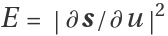,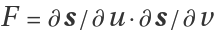and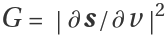.
The line element ds can be expressed in terms of the coefficients of the first fundamental form as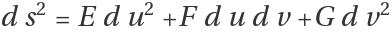and determines the arc length of a curve on a surface.
The first fundamental form can be written as the metric tensor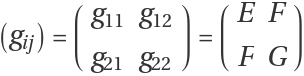.
In orthogonally curvilinear coordinates, F=0.
This is useful in the calculation of curvature and intrinsic properties of a surface such as length and area.

## Examples

### Basic Examples (2)

Get the first fundamental form of a sphere:

 In:=Out=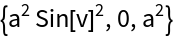In:=Out=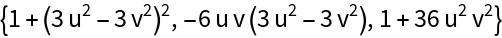Enrique Zeleny

## Version History

• 2.0.0 – 23 July 2020
• 1.0.0 – 08 April 2020